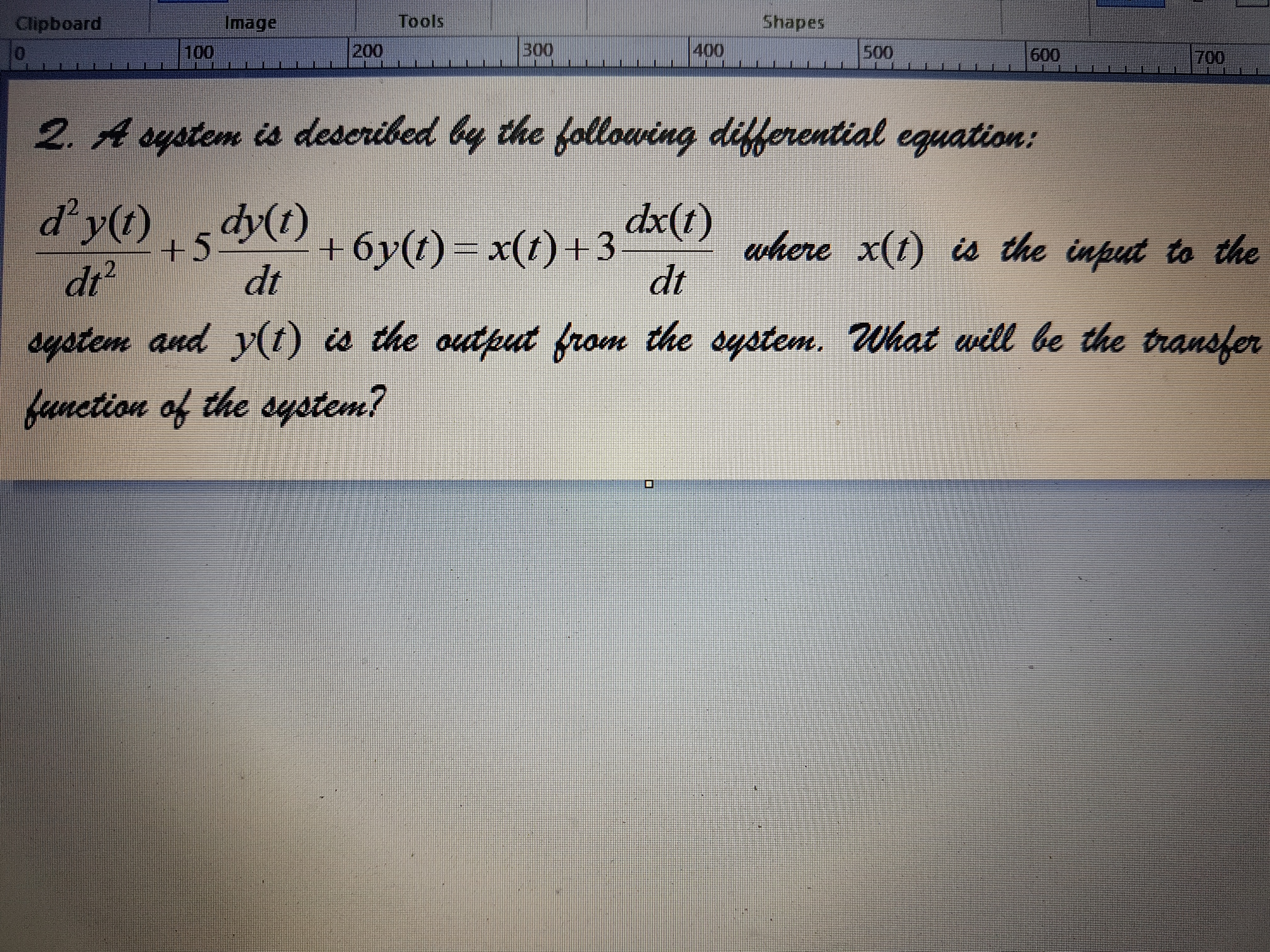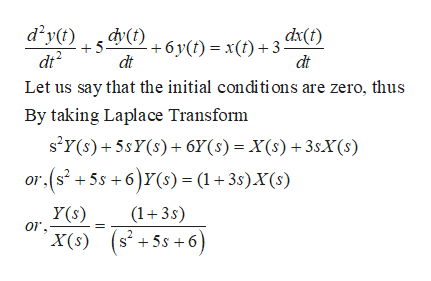# ImageShapesToolsClipboard100400В0020050007006002. Aoystem is deseribed by the following diflerential equation:dx(t)where x(t) is the input to thedtd'y)drsystem and y(t) is the output from the oystem. What will be the transferdy(t)+6y(t) x(t) +3+5)dtfunetion of the dystem!

Question
1 views

Solve the following question in detail:help_outlineImage TranscriptioncloseImage Shapes Tools Clipboard 100 400 В00 200 500 0 700 600 2. Aoystem is deseribed by the following diflerential equation: dx(t) where x(t) is the input to the dt d'y) dr system and y(t) is the output from the oystem. What will be the transfer dy(t) +6y(t) x(t) +3 +5) dt funetion of the dystem! fullscreen
check_circle

Step 1

Taking laplace t...help_outlineImage Transcriptionclosed y(t) dy(t) dx(t) 5. 6 y (t)= x(f)3 dt dt Let us say that the initial conditions are zero, thus By taking Laplace Transform s'Y(s)+5sY(s)6Y(s) = X(s)+3sX(S) r.(s2 +5s +6)Y(s) = (1+3s)X(s) Y(s) or (13s) X(s) s+5s+6 2 fullscreen

### Want to see the full answer?

See Solution

#### Want to see this answer and more?

Solutions are written by subject experts who are available 24/7. Questions are typically answered within 1 hour.*

See Solution
*Response times may vary by subject and question.
Tagged in

### Electrical Engineering